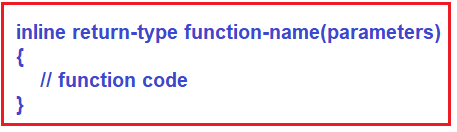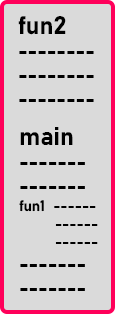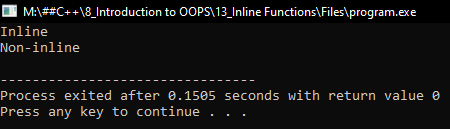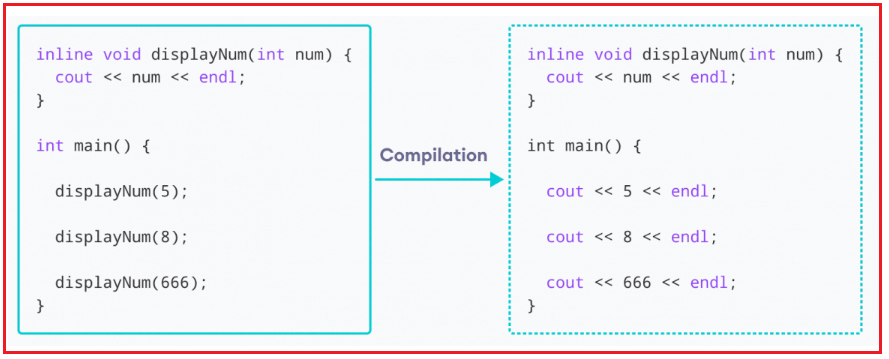# Inline Functions in C++

## Inline Functions in C++ with Examples:

In this article, I am going to discuss Inline Functions in C++ with Examples. Please read our previous article where we discussed Scope Resolution Operator in C++ with Examples. The Inline function is one of the important features of C++.

##### What is Inline Function?

Normally, a function call transfers the control from the calling program to the called function. This process of jumping to the function, saving registers, passing a value to the argument, and returning the value to the calling function takes extra time and memory.

An inline function is a function that is expanded inline when it is invoked, thus saving time. The compiler replaces the function call with the corresponding function code, which reduces the overhead of function calls.

When an inline function is called, the compiler replaces all the calling statements with the function definition. Every time an inline function is called, the compiler generates a copy of the function’s code, in place, to avoid the function call.

##### Why inline functions are used and what is the purpose of inline functions?

Now, let us understand why and when we should use inline functions in C++. The main use of the inline function in C++ is to save memory space. Whenever a function is called, then it takes a lot of time to execute the tasks, such as moving to the calling function. If the length of the function code is very small and if takes a very small amount of time to execute, then sometimes the time taken required for moving to the calling function will be greater than the time taken required to execute that function. The solution to this problem is to use Inline Functions.

In the case of function calls, the time for calling small functions is huge, so to overcome such a problem, C++ Introduced a new feature called Inline Function. When the function is called inside the main() method, it is expanded with its definition thus saving time.

C++ provides an inline function to reduce the function call overhead. An inline function is a function that is expanded in line when it is called. When the inline function is called whole code of the inline function gets inserted or substituted at the point of the inline function call. This substitution is performed by the C++ compiler at compile time. The inline function may increase efficiency if it is small. The syntax for defining the function inline is:##### Example to Understand Inline Functions in C++:

The functions which expand in the same line where they are called are Inline Functions in C++. There is no separate block for that function. For a better understanding of the Inline Function, please have a look at the below code.

```class Test
{
public:
void fun1 ()
{
cout << "Inline";
}
void fun2 ();
};

void Test::fun2()
{
cout << "Non-inline";
}

int main()
{
Test t;
t.fun1();
t.fun2();
}
```

Here we have a class Test which is having two functions fun1() and fun2(). We have done the body implementation of fun1() inside the class and of fun2() outside the class using the scope resolution operator. Here we have two types of functions that are outside the class and inside the class.

Then we have written the main function and we have created an object t of class Test. Then we are calling those two functions i.e. ‘un1() and fun2(). Now if you look at the machine code or object code generated for this code then how does it look like? Let us see.The above image shows the object code for our program. Let us assume that dotted lines are the machine instructions. Then there is a call for fun1 inside the main function. And for fun2 separate machine code will be generated.

So, what do you find? The machine code of function fun1 is written at the place of function call inside the main function. When you see the execution of the main function, fun1 will execute as a part of the main. So, what has happened here? fun1 function has expanded inline. So that is the meaning of the inline function. The function’s machine code will be pasted or copied at the place of the function call. So fun1 is an inline function.

Now, how do make a function inline? If you define a function inside a class automatically it is inline. And if you write a function outside the class then automatically that is a non-inline function. If you want to make a function non-inline then you must write that function outside the class. If you want to make a function inline then you must write that function inside the class.

There is one more method to make a function inline and in that case, we just need to write inline before the function. So, to make fun2 inline, we need to declare the function inside the class as follows:

inline void fun2();

We have just added the inline keyword before the function return type inside the class. This will become an inline function and the code will not be separately generated but it is copied inside the main function. That’s all about inline function. Now let us write the complete program.

##### Example to Understand Inline Functions in C++:
```#include <iostream>
using namespace std;
class Test
{
public:
void fun1()
{
cout << "Inline\n";
}
inline void fun2();
};

void Test::fun2()
{
cout << "Non-inline\n";
}

int main()
{
Test t;
t.fun1();
t.fun2();
}
```
###### Output:##### Where we cannot use Inline Functions?

Remember, making a function inline is only a request to the compiler, not a command. So, the C++ Compiler can ignore the request for inlining. The compiler may not perform inlining to the functions in the following circumstances

1. If the function is a recursive function.
2. If the function contains a loop like for, while, do-while loop.
3. If the function contains static variables.
4. If the function contains a switch or goto statement.
5. If the function return type is other than void, and the return statement doesn’t exist in the function body.
##### When do we require an Inline Function in C++?

In C++, we can use the inline function in the following scenarios:

1. We can use the inline function to improve the application performance.
2. It can be used to overcome the macros.
3. We can use the inline function outside the class so that we can hide the internal implementation of the function.
##### Advantages of Inline functions in C++:

Following are the advantages of using Inline Functions in C++.

1. Function call overhead doesn’t occur. In the inline function, we do not require to call a function, so it does not cause any overhead.
2. It also saves the overhead of the return statement from a function.
3. It does not require any stack on which we can push or pop the variables as it does not perform any function calls. So, it saves the overhead of push/pop variables on the stack when the function is called
4. An inline function is mainly beneficial for the embedded systems as it yields less code than a normal function.
##### Disadvantages of Inline Function in C++:
1. The variables that are created inside the inline function will consume additional registers. After the in-lining function, if the variable number which is going to use the register increases, then the use of registers also increases, which may increase the overhead on register variable resource utilization. It means that when the function call is replaced with the inline function body, the total number of variables used by the function also gets inserted. So the number of registers going to be used for the variables will also get increased. This causes an overhead on resource utilization.
2. If we use many inline functions, then the binary executable file also becomes large because of the duplication of the same code.
3. The use of too many inline functions can reduce the instruction cache hit rate, thus reducing the speed of instruction fetch from the cache memory to that of the primary memory.
4. It also increases the compile-time overhead because if someone changes the code inside the inline function, then the code needs to be recompiled again to reflect the changes; otherwise, it will execute the old functionality.
5. Inline functions might cause thrashing due to the increase in the size of the binary executable file. Thrashing in memory causes the performance of the computer to degrade.
##### Working of inline functions in C++:As shown in the above image, we have created an inline function named displayNum() that takes a single integer as a parameter. We then called the function 3 times inside the main() function with different arguments. Each time displayNum() is called, the compiler copies the code of the function to that call location.